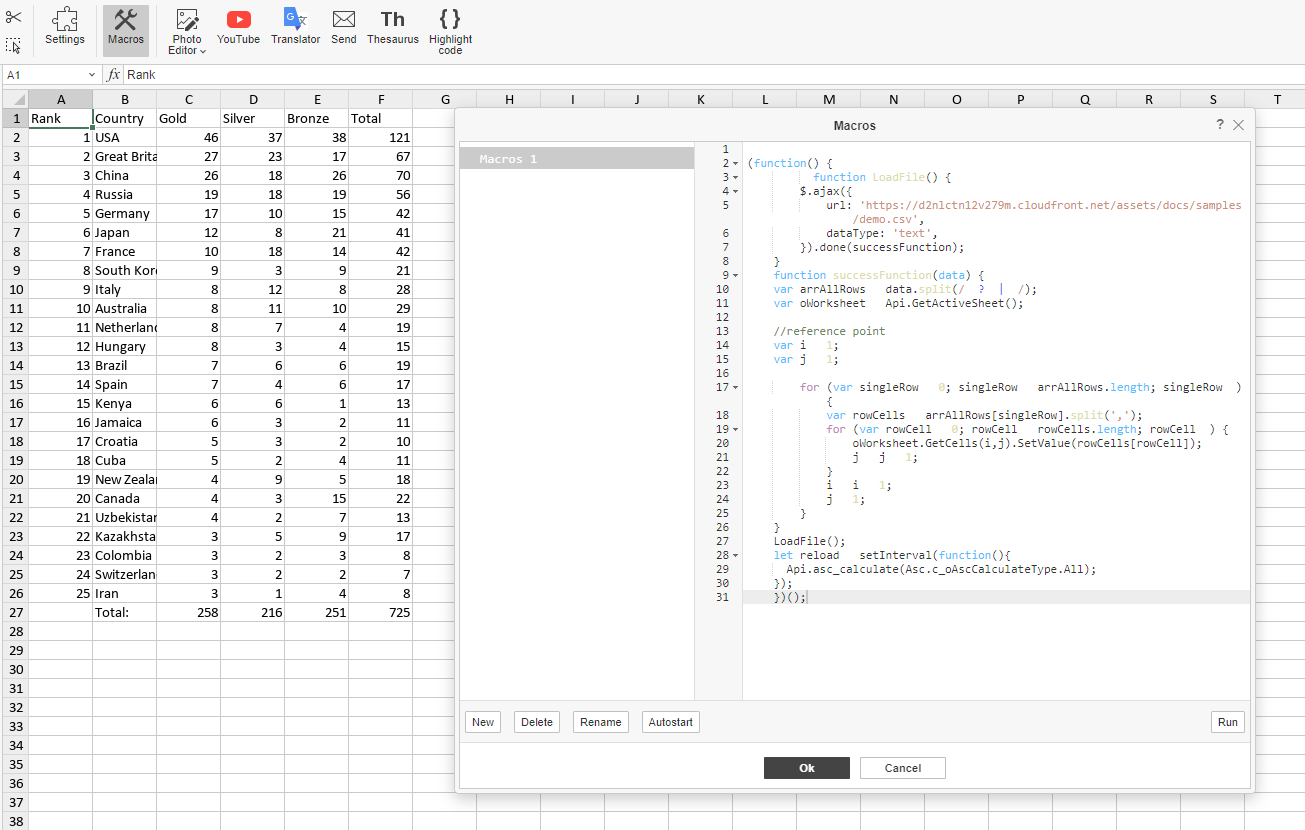# Use ONLYOFFICE macro to import CSV and TXT data into your spreadsheet

18 October 2022

Storing tabular data in a CSV format is very practical in many ways, which makes this type of file extremely popular. ONLYOFFICE Docs allow importing local CSV and TXT files. However, what if we want to import these files from the web without having to download them? This blog post will share a little trick on how it can be done.In our previous posts, we talked about ONLYOFFICE macros. They are JavaScript based, and we can’t stress enough how versatile they are. This feature will help us tackle importing remote CSV and TXT files. So, as you might have already guessed, we are going to create another macro.

Note! This macro is only operational with the desktop version of ONLYOFFICE Docs.

## Building a macro

First, we need to fetch the file. JavaScript is the cornerstone of web development, and it has all the necessary tools already built in. In our case, we are going to use AJAX request:

``````      function LoadFile() {
\$.ajax({
dataType: 'text',
}).done(successFunction);
}
}
``````

Now we have the data, but it’s raw, so we need to format it. First, we split it into lines:

`````` function successFunction(data) {
var arrAllRows = data.split(/\r?\n|\r/);
``````

Then we get the active spreadsheet:

``````var oWorksheet = Api.GetActiveSheet();
``````

We have already split the array data into lines, and now we split it into separate words:

``````   for (var singleRow = 0; singleRow < arrAllRows.length; singleRow++) {
var rowCells = arrAllRows[singleRow].split(',');
}
``````

Then we insert these values into cells. To do that, we will use the GetCells and SetValue methods. The first one selects the range, and the latter inserts the value into the selected cell. The GetCells method takes two parameters: the number of rows and the number of columns. To indicate the number of rows and columns, we will use i and j variables and increment them at every for-loop iteration:

`````` for (var rowCell = 0; rowCell < rowCells.length; rowCell++) {
oWorksheet.GetCells(i,j).SetValue(rowCells[rowCell]);
j = j + 1;
}
i = i + 1;
j = 1;
}
}
``````

And finally, in order to display the new values, we need to recalculate the entire document. We will do it by implementing the Api.asc_calculate method. We used this method in our Air Quality macro. It allowed us to recalculate the incoming data with a certain interval. But this time, we will remove the interval parameter. This way, the document will be recalculated instantly:

``````  let reload = setInterval(function(){
Api.asc_calculate(Asc.c_oAscCalculateType.All);
});
``````

The entire macro code is the following:

``````(function() {
\$.ajax({
dataType: 'text',
}).done(successFunction);
}
function successFunction(data) {
var arrAllRows = data.split(/\r?\n|\r/);
var oWorksheet = Api.GetActiveSheet();

//reference point
var i = 1;
var j = 1;

for (var singleRow = 0; singleRow < arrAllRows.length; singleRow++) {
var rowCells = arrAllRows[singleRow].split(',');
for (var rowCell = 0; rowCell < rowCells.length; rowCell++) {
oWorksheet.GetCells(i,j).SetValue(rowCells[rowCell]);
j = j + 1;
}
i = i + 1;
j = 1;
}
}